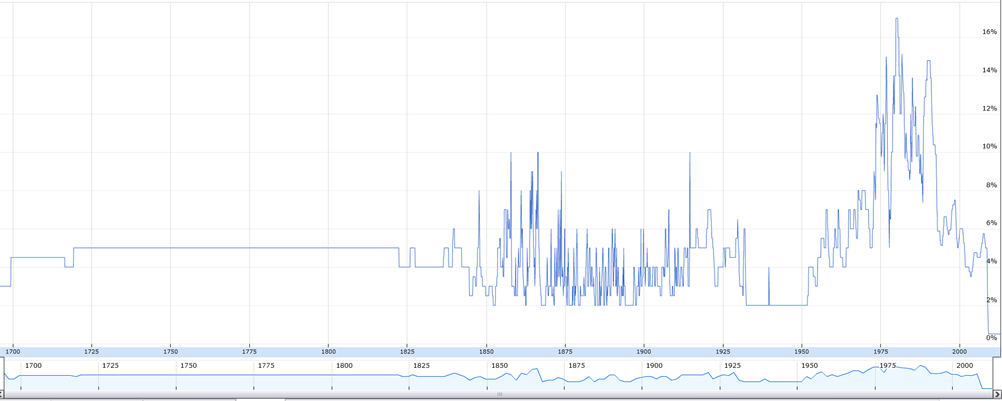# How Abnormal Are Rates?

The Guardian of U.K. has Bank of England’s base interest rates data from the year 1694, which gives us some interesting things to ponder over.

At present the base rate is 0.50%. This is how the interest rates have varied over 318 yeas – from 1694 to 2012.

• Interest rate less than 1.00% = four years or 1% of total span
• Interest  rates between 1 and 2% = 0 years or 0% of total span
• Interest  rates between 2 and 3% = 40 years or 13% of total span
• Interest  rates between 3 and 4% = 44 years or 14% of total span
• Interest  rates between 4 and 5% = 63 years or 20% of total span
• Interest  rates between 5 and 6% = 131 years or 41% of total span
• Interest  rates over 6% = 36 years or 11% of total span

The average base rate of al years is 4.85%. Average by centuries are

• 1700 = 4.89%
• 1800 = 4.06%
• 1900 = 5.53%